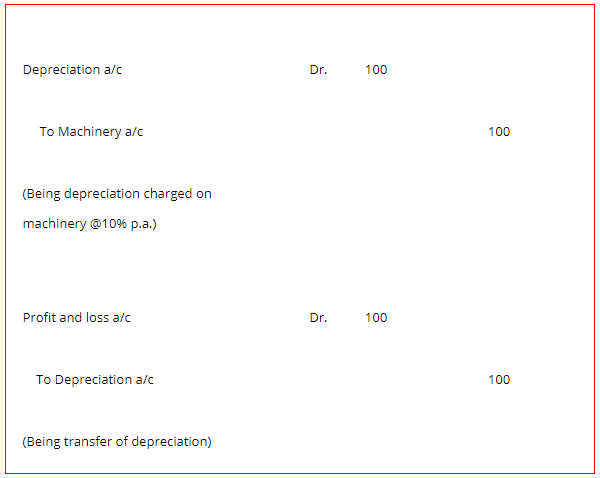# Depreciation Accounting: Direct and Indirect Method Accounting Treatment

The compilation of these Depreciation, Provision and Reserves Notes makes students exam preparation simpler and organised.

## Methods of Recording Depreciation

Depreciation is an integral component of accounting. It stands to impact the preparation of accounts. The amount recorded under the head of depreciation ultimately impacts the amount shown as profit or loss in the statement of income. Hence, it is pertinent to study and make calculations for the same in a calculated manner, which ensures a fair and accurate presentation of accounts. Let us study the methods of recording depreciation as per depreciation accounting.

### Methods of Depreciation Accounting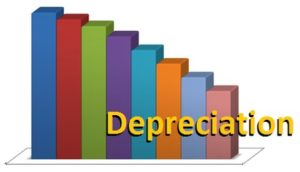Usually, there are two methods of recording depreciation in depreciation accounting. They are as follows:

Direct Method (No Provision for Depreciation Account is Maintained)
You can charge depreciation by debiting the Depreciation Account and crediting the respective Asset Account. Further, close the Depreciation account by transferring the amount to the Profit and Loss Account at the end of the year. The asset account then appears in the Balance Sheet at its written down value that is, cost less depreciation at the end of the year.

Let’s take a look at the journal entries that must be recorded under this method of depreciation accounting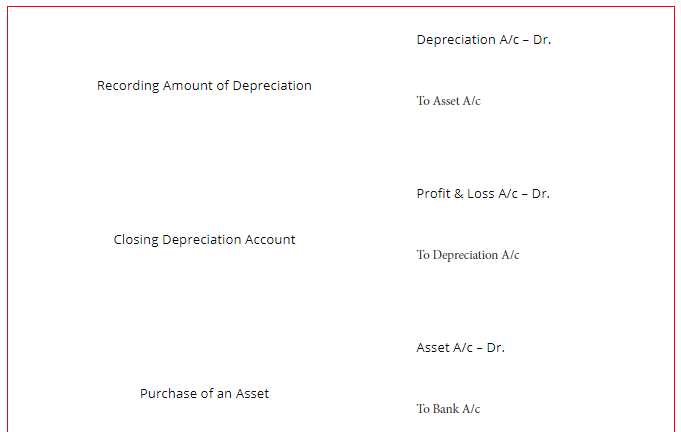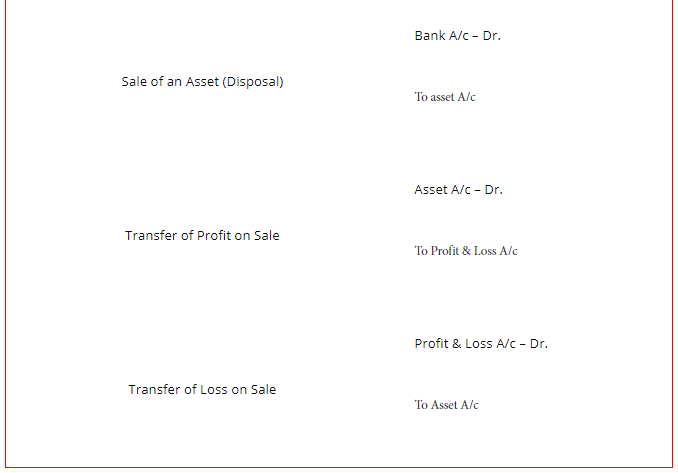Indirect Method (Provision for Depreciation Account is Maintained)
You have to debit the amount of depreciation to the Depreciation Account and credit it to the Provision for Depreciation Account (or Accumulated Depreciation Account, if so maintained). The amount of depreciation is then trans­ferred to the Profit and Loss Account at the end of the year. However, the Asset Account will appear at a cost.

Further, the accumulated depreciation appears either shown as a deduction from the asset or the same may appear in the liability side of the Balance Sheet. Let’s take a look at the journal entries that are different from the direct method. The other entries will remain the same.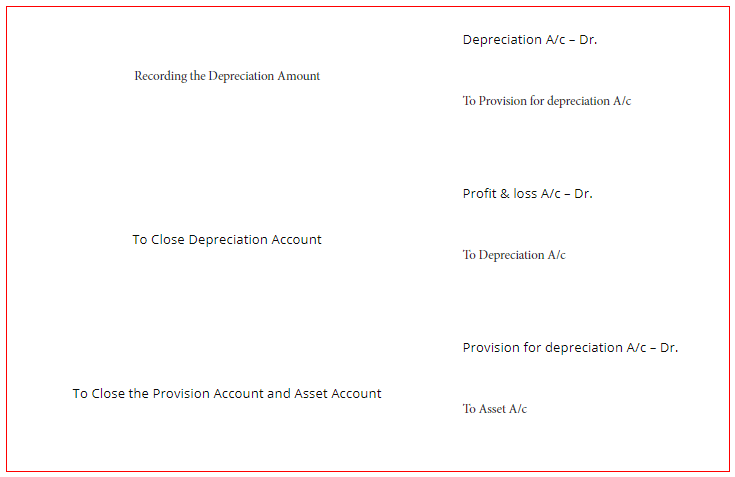Take note that the profit and loss on the sale of the asset will remain the same.

Example:

Question 1.
The cost of a machine is IR 1,000 and its depreciation is 10% p.a. show the entries to record the depreciation.